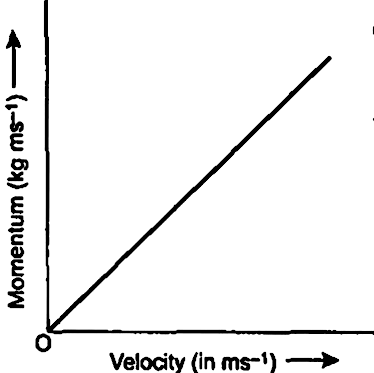Home/Class 9/Physics/

What is momentum? Write its $$SI$$ unit. Interpret force in terms of momentum. Represent the following graphically : momentum versus velocity when mass is fixed.Speed
00:00
05:18## QuestionPhysicsClass 9

What is momentum? Write its $$SI$$ unit. Interpret force in terms of momentum. Represent the following graphically : momentum versus velocity when mass is fixed.

Momentum is measured as product of mass and velocity.
Momentum $$=$$ mass $$×$$ velocity
$$p=m (kg)\times v(ms^{-1})$$
$$SI$$ unit of momentum is $$kg\ m\ s^{-1}$$
Force is defined as rate of change of momentum. Let an object of mass $$m$$ move with initial velocity $$u$$ and after some time the velocity changes to $$v$$. Thus $$F=mv-mu$$
$$F=p_2-p_1$$
The graph between momentum and velocity is shown below. When $$m$$ is fixed, momentum is given as $$p\propto v$$.